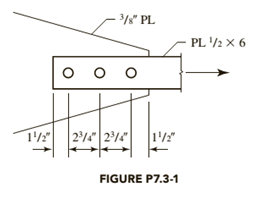# The tension member is a PL 1 2 × 6 . It is connected to a 3 8 -inch-thick gusset plate with 7 8 -inch-diameter bolts. Both components are of A36 steel. a . Check all spacing and edge-distance requirements. b . Compute the nominal bearing strength of each bolt.### Steel Design (Activate Learning wi...

6th Edition
Segui + 1 other
Publisher: Cengage Learning
ISBN: 9781337094740### Steel Design (Activate Learning wi...

6th Edition
Segui + 1 other
Publisher: Cengage Learning
ISBN: 9781337094740

#### Solutions

Chapter
Section
Chapter 7, Problem 7.3.1P
Textbook Problem

## The tension member is a PL 1 2 × 6 . It is connected to a 3 8 -inch-thick gusset plate with 7 8 -inch-diameter bolts. Both components are of A36 steel.a. Check all spacing and edge-distance requirements.b. Compute the nominal bearing strength of each bolt.Expert Solution
To determine

(a)

If all the spacing and edge distance requirements are adequate.

The spacing and the edge distance provided are adequate.

### Explanation of Solution

Given:

Tension member is PL12×6.

Thickness of the gusset plate is 38inch.

Diameter of the bolts is 78inch.

Steel Used is A36.

Calculation:

The properties for A36 steel from the AISC steel table are as follows:

The ultimate tensile stress is 58ksi.

Write the expression for minimum spacing.

Sm=83d ...... (I)

Here, diameter of the bolt is d and minimum spacing is Sm.

Substitute 78inch for d in Equation (I).

Sm=83(78inch)=2.33inch

The minimum spacing between the bolts is 2.33inch which is less than the actual spacing between the bolts, that is 2.75inch. Therefore, the spacing is adequate.

For the edge distance to be adequate, the value of edge distance should be greater than or equal to the value of minimum edge distance.

lelm

Here, edge distance is le and minimum edge distance is lm.

The value of edge distance is 1.5inch and the value of minimum edge distance is 1.5inch, which are equal. Therefore, the edge distance provided is adequate.

Conclusion:

Thus, the spacing and the edge distance provided are adequate.

Expert Solution
To determine

(b)

The nominal bearing strength of each bolt.

The nominal bearing strength of the edge bolt is 26.91kips and the nominal bearing strength for inner bolts is 45.68kips.

### Explanation of Solution

Calculation:

Write the expression for nominal bearing strength of a bolt.

Rn=1.2lctFu ...... (II)

Here, the nominal bearing strength is Rn, thickness is t, ultimate tensile stress is Fu, and the distance between the edge of the adjacent hole to the edge of the bolt hole is lc.

With the upper limit of nominal bearing strength of the bolt,

Rn=2.4dtFu

Write the expression to calculate the value of lc for edge bolts.

lc=leh2 ...... (III)

Here, height of the bolt is h.

Write the expression to calculate the value of lc for inner bolts.

lc=sh ...... (IV)

Here, spacing between the bolts is s.

Write the expression to calculate the height of the bolt.

h=d+116inch ...... (V)

Calculate the value of h.

Substitute 78inch for d in Equation (V).

h=78inch+116inch=1516inch

Calculate the value of lc for edge bolts.

Substitute 1.5inch for le and 1516inch for h in Equation (III).

lc=1.5inch(1516inch)2=1.031inch

Calculate the nominal bearing strength for edge bolts.

Substitute 1.031inch for lc, 38inch for t and 58ksi for Fu in Equation (II).

Rn=1.2(1.031inch)(38inch)(58ksi)=26.91kips

Calculate the upper limit of nominal bearing strength for edge bolts.

Substitute 78inch for d, 38inch for t and 58ksi for Fu.

Rn=2.4(78inch)(38inch)(58ksi)=45.68kips

Thus, the minimum value of Rn is considered and is equal to 26.91kips.

Calculate the value of lc for inner bolts.

Substitute 2.75inch for s and 1516inch for h in Equation (IV).

lc=2.75inch1516inch=1.813inch

Calculate the nominal bearing strength for inner bolts.

Substitute 1.813inch for lc, 38inch for t and 58ksi for Fu in Equation (II).

Rn=1.2(1.813inch)(38inch)(58ksi)=47.32kips

Calculate the upper limit of nominal bearing strength for inner bolts.

Substitute 78inch for d, 38inch for t and 58ksi for Fu.

Rn=2.4(78inch)(38inch)(58ksi)=45.68kips

Thus, the minimum value of Rn is considered and is equal to 45.68kips.

Conclusion:

Thus, the nominal bearing strength of the edge bolt is 26.91kips and the nominal bearing strength for inner bolts is 45.68kips.

### Want to see more full solutions like this?

Subscribe now to access step-by-step solutions to millions of textbook problems written by subject matter experts!

Get Solutions

### Want to see more full solutions like this?

Subscribe now to access step-by-step solutions to millions of textbook problems written by subject matter experts!

Get Solutions

Find more solutions based on key concepts
Show solutions
Discuss in detail at least two concepts (for example, activities, processes, or methods) that can be employed d...

Engineering Fundamentals: An Introduction to Engineering (MindTap Course List)

What is the purpose of NFPA and HMIS labeling?

Precision Machining Technology (MindTap Course List)

What are control relays?

Electric Motor Control

What enterprise resource planning (ERP)? What is supply chain management (SCM)?

Systems Analysis and Design (Shelly Cashman Series) (MindTap Course List)

Compare and contrast the different methods of obtaining help. Which method do you think is the best? Why?

Enhanced Discovering Computers 2017 (Shelly Cashman Series) (MindTap Course List)

If your motherboard supports ECC DDR3 memory, can you substitute non-ECC DDR3 memory?

A+ Guide to Hardware (Standalone Book) (MindTap Course List)